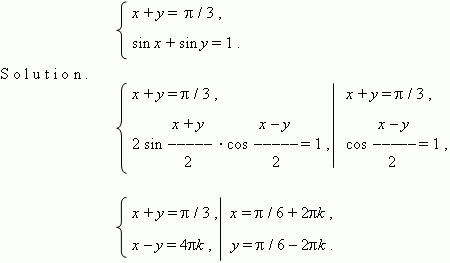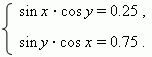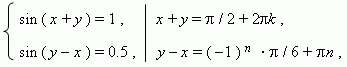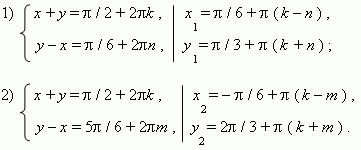# Systems of simultaneous trigonometric equations

At solving simultaneous trigonometric equations we use the same methods, as in algebra ( exchange, substitution, elimination etc.) and also the known methods and formulas of trigonometry. Consider some examples.

E x a m p l e  1 .   Solve the system of simultaneous equations:E x a m p l e   2 .  Solve the system of simultaneous equations:S o l u t i o n .  Adding and subtracting these two equations, we receive:Consider each of the branches of the second equation solution separately: